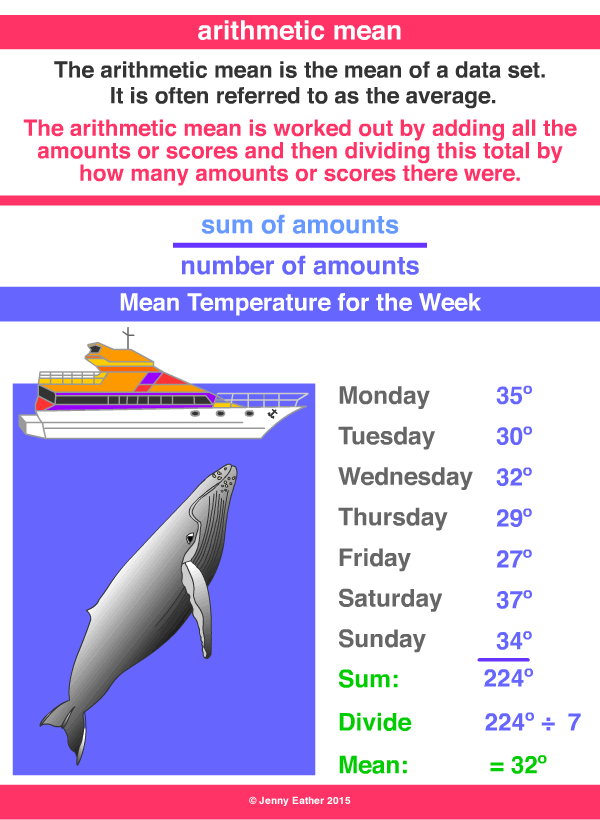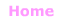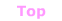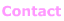Aa Bb Cc Dd Ee Ff Gg Hh Ii Jj Kk Ll Mm Nn Oo Pp Qq Rr Ss Tt Uu Vv Ww Xx Yy Zz

Aa

arithmetic mean

• in statistics, the mean of a data set,
often referred to as the average.

to calculate the arithmetic mean
1. Add up all the values.
2. Divide by the number of values.

EXAMPLES:© Jenny Eather 2014. All rights reserved.